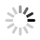# 大厂硬核干货！深入分析弹性动效的应用及原理

#### 1. 在原型工具中设计弹性动效

1. Origami Studio2. Principle3. 其他△ ProtoPie Studio△ Flinto

#### 2. 在主流平台实现弹性动效

1. iOS

UIView.animateWithDuration:usingSpringWithDamping:initialSpringVelocity + (void)animateWithDuration:(NSTimeInterval)duration delay:(NSTimeInterval)delay usingSpringWithDamping:(CGFloat)dampingRatio initialSpringVelocity:(CGFloat)velocity options:(UIViewAnimationOptions)options animations:(void (^)(void))animations completion:(void (^)(BOOL finished))completion;

• dampingRatio：与弹性动效静止时的阻尼比。值为 1 时将得到平稳减速没有弹性的效果（即临界阻尼状态），值越接近 0 震荡（弹性）程度越大。velocity：弹性动效的初始速度。为了平滑地开始动效，请把这个值与之前附着的视图的速度匹配。

* 注：iOS 系统常用的临界阻尼效果，参数为：duration: 0.5,dampingRatio: 1

UIViewPropertyAnimator 的参数中提到：

The damping ratio for the spring is computed from the formula damping / (2 * sqrt (stiffness * mass)).

—— UISpringTimingParameters

2. Android

findViewById(R.id.imageView).also { img -> SpringAnimation(img,DynamicAnimation.TRANSLATION_X).apply { … spring.dampingRatio = SpringForce.DAMPING_RATIO_LOW_BOUNCY spring.stiffness = SpringForce.STIFFNESS_LOW setStartVelocity(velocity) } }

SpringAnimation 提供了 3 个参数来定义弹性动效：dampingRatio、stiffness 和 setStartVelocity。

setStartVelocity：起始速度用于定义在动画开始时动画属性更改的速度。

dampingRatio：阻尼比用于描述弹簧震动逐渐衰减的状况。通过使用阻尼比，您可以定义震动从一次弹跳到下一次弹跳所衰减的速度有多快。以下列出了可使弹簧弹力衰减的四种不同方式：

• 当阻尼比大于 1 时，会出现过阻尼现象。它会使对象快速地返回到静止位置。
• 当阻尼比等于 1 时，会出现临界阻尼现象。这会使对象在最短时间内返回到静止位置。
• 当阻尼比小于 1 时，会出现欠阻尼现象。这会使对象多次经过并越过静止位置，然后逐渐到达静止位置。
• 当阻尼比等于零时，便会出现无阻尼现象。这会使对象永远震动下去。

stiffness：刚度定义了用于衡量弹簧强度的弹簧常量。不在静止位置的坚硬弹簧可对所连接的对象施加更大的力。

3. Web

easing: 'spring(mass,stiffness,damping,velocity)'

#### 3. 从设计到实现的差异

1. 设计工具2. 开发平台3. 差异

#### 4. 弹性系统原理

1. 什么是弹性系统

2. 质量（Mass）3. 阻尼（Damping）

4. 阻尼比（Damping Ratio）

• 无阻尼：阻尼比 -> 0，系统处于永远震荡的状态；
• 欠阻尼：阻尼比 < 1，对象进行指数递减的震荡运动；
• 过阻尼：阻尼比 > 1，对象进行无震荡的减速运动；
• 临界阻尼：阻尼比 = 1，对象以最短时间结束运动。5. 刚度（Stiffness）6. 摩擦力（Friction）

7. 张力（Tension）

#### 结论与成果

damping = friction stiffness = tension [default]mass = 1 dampingRatio = damping / (2 * sqrt (mass * stiffness)) [default]velocity = 0

1. 应用

2. Origami

• Bounciness = 5
• Speed = 10

• Friction = 27.0487
• Tension = 299.61884

• damping = 27.05
• stiffness = 299.62
• mass = 1
• velocity = 0
• dampingRatio = 0.78

For Android

SpringAnimation(object,DynamicAnimation.[property]).apply { … spring.dampingRatio = 0.78f spring.stiffness = 299.62f setStartVelocity(0f) }

For iOS

let spring = CASpringAnimation(keyPath:[property]) spring.stiffness = 299.62 spring.damping = 27.05 spring.mass = 1 spring.initialVelocity = 0

3. Principle

• Tension = 381.47
• Friction = 20.17

• damping = 20.17
• stiffness = 381.47
• mass = 1
• velocity = 0
• dampingRatio = 0.52

For Android

SpringAnimation(object,DynamicAnimation.[property]).apply { … spring.dampingRatio = 0.52f spring.stiffness = 381.47f setStartVelocity(0f) }

For iOS

let spring = CASpringAnimation(keyPath:[property]) spring.stiffness = 381.47 spring.damping = 20.17 spring.mass = 1 spring.initialVelocity = 0

4. UIViewSpring 的 duration 参数

Framer Studio 可以默认使用 time 与 damping 定义弹性动效，在弹性动效的代码区域，右键菜单可以看到 Copy Animation 中包含 2 个选项，复制 Damping and Duration 的值，可以看到在 Framer Studio 中用于定义弹性动效的默认方法中：time 对应的是 duration，damping 对应的是 dampingRatio。# Tries to compute the duration of a spring,# but can't for certain velocities and if dampingRatio >= 1 # In those cases it will return null ePSilon = 0.001 computeDuration = (tension,friction,velocity = 0,mass = 1) -> dampingRatio = computeDampingRatio(tension,friction) undampedFrequency = Math.sqrt(tension / mass) # This is basically duration extracted out of the envelope functions if dampingRatio < 1 a = Math.sqrt(1 - Math.pow(dampingRatio,2)) b = velocity / (a * undampedFrequency) c = dampingRatio / a d = - ((b - c) / ePSilon) if d <= 0 return null duration = Math.log(d) / (dampingRatio * undampedFrequency) else return null return duration 引用自：SpringCurveValueConverter.coffee host with ❤️Github

5. 转换器6. Origami Patch#### 插值模拟

1. 自定义动态插值器import android.view.animation.BaseInterpolator; public class SpringInterpolator extends BaseInterpolator{ private float mFactor; public SpringInterpolator() { this.mFactor = 0.5f; } public SpringInterpolator(float mFactor) { this.mFactor = mFactor; } @Override public float getInterpolation(float input) { if (input == 0.0f || input == 1.0f) return input; else { float value = (float) (Math.pow(2,-10 * input) * Math.sin((input - mFactor / 4.0d) * (2.0d * Math.PI) / mFactor) + 1); return value; } } }

SpringInterpolator 进行不同 factor 的最大值的遍历与匹配，最终得出一个近似效果对应的 factor 值。由于需要大量的重复计算，且没有通用公式，此方法仅被应用在 Web 转换器中。

2. 自定义 XML 插值器#### 总结

• 谐振子系统：httPS://en.wikipedia.org/wiki/Harmonic_oscillator
• 胡克定律：httPS://en.wikipedia.org/wiki/Hooke%27s_law
• 阻尼比：httPS://en.wikipedia.org/wiki/Damping_ratio
• 刚度：httPS://en.wikipedia.org/wiki/Stiffness
• 张力：httPS://en.wikipedia.org/wiki/Tension_(physics)
• FramerJS：httPS://github.com/koenbok/Framer# 猜你在找的UIUI动效现如今在 APP 和网页中几乎已经成为了基本的组成部分，经过仔细打磨的 UI动效对于整个界面的提升是显著的。10个动效案例，让大家避开动效误区。 一、走路 在做走路动画的时候，除了要把脚与地面的接触做出来，更应该要注意的是身体不同部位的跟随与节奏。